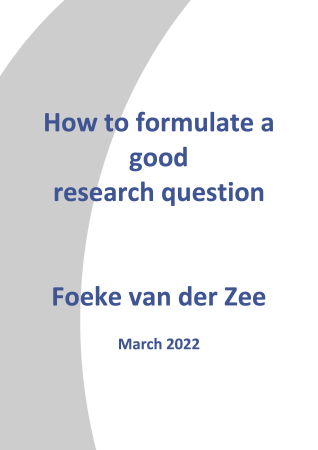Model# Research-Coaches.com

## We show you the way how to conduct perfect research

### A model is a replica of the empery (the real world). Some models are an exact replica, but most of the time the model is a simplification of the empery.

Models are used for explaining how the real world is functioning. Several types of models can be distinguished. They are described below. Though different types of models can be distinguished, most of the time the combination of a linguistic and a visual model are used together to explain things in a clear way. Sometimes this is called a theory. Theories however are slightly different from the combination of models. You can read more about this issue on our page called theory.

## Model as an exact replica

This type of models are often used in medicine. A replica of the human body with several parts that can be taken out and put together again. They are useful in explaining where the body parts are. To explain the function of every part, words are needed.

An exact replica can be a one-to-one replica. It is often more useful to shrink the empery into a smaller replica (for example a building), or to blow it up to a large one (for example the structure of an atom).

## Model as a graphical model

This is a drawing. The advantage of this type of model compared to a replica is that it isn’t three dimensional so it can be used in textbooks or on websites. Written words can be used to give a full explanation.

The aspects of the real world can be represented in the graphic model in a concrete but also very abstract way. The more abstracted the display, the more redundant the model.

## Model as a linguistic model

In a linguistic model everything is explained in words. It is hard to explain it clearly so most of the time these linguistic models are combined with the other types of models.

## Model as a mathematical model

This type of models reduce the empery to an arithmetic operation. 2 + 2 = 4 might mean two sheep and two sheep are four sheep. It can also mean two men and two men are four men.

Statistics is based on mathematical models. Sometimes the term model is explicitly used, for instance in regression.

## Conceptual models

A conceptual model is an abstracted model for a research. It explains which parts of the empery are measured and how these aspects – according to a theory – are related. Conceptual models are tested with statistics. They are useful for making a theory or to test a theory.

## Final remarks

A model is always redundant to the empery. This means, not every aspect of the empery is out of necessity represented in the model. Most of the time the aspects that don’t help to clarify the real world are left out.

## Related topics to model: